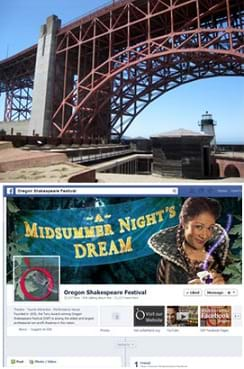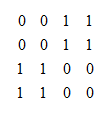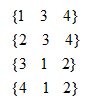# LessonGraph Theory in Drama

(0 Ratings)

### Quick Look

Grade Level: 11 (9-12)

Time Required: 30 minutes

Lesson Dependency: None

Subject Areas: Computer Science, Data Analysis and ProbabilityStructural engineers use graph theory to represent the forces in a truss. Software engineers use graph theory to understand relationships between people, such as those in social networks like Facebook.

### Summary

Students use graph theory to create social graphs for their own social networks and apply what learn to create a graph representing the social dynamics found in a dramatic text. Students then derive meaning based on what they know about the text from the graphs they created. Students learn graph theory vocabulary, as well as engineering applications of graph theory.

### Engineering Connection

Graph theory is the study of graphs and is applicable in computer science, mathematics and engineering. A graph is a mathematical structure used to model relationships between the objects in a set of objects. Graphs in this context have vertices or "nodes" and a group of edges, which connect pairs of vertices.

In computer science, software engineers use graphs to represent communication networks, data organization, computational devices, the flow of computation and more. One example of graph theory used by computer/software engineers is the multi-hop cooperative relay communication networks in which the network is comprised of a base station(s) and relay stations (nodes) that communicate information to mobile communication devices. Another is a social network that software engineers are exploiting on sites like Facebook to market products to consumers. Software engineers can use graph theory to represent the structure of a website. The vertices are the available web pages and the edges are the connections (directional) between pages.

In the design of integrated circuits (ICs) for electronic devices, electrical engineers and computer electronic engineers also employ graph theory. ICs have complex layered microcircuits that can be represented as nodes interconnected by lines or arcs. By using graph theory, engineers can optimize the density of components and minimize the connections to optimize processing speed and electrical efficiency.

### Learning Objectives

After this lesson, students should be able to:

• Explain the basic concepts of graph theory.
• Apply graph theory to the analysis of a network.
• Explore the practical concepts of graph theory through questioning.

### Educational Standards Each TeachEngineering lesson or activity is correlated to one or more K-12 science, technology, engineering or math (STEM) educational standards. All 100,000+ K-12 STEM standards covered in TeachEngineering are collected, maintained and packaged by the Achievement Standards Network (ASN), a project of D2L (www.achievementstandards.org). In the ASN, standards are hierarchically structured: first by source; e.g., by state; within source by type; e.g., science or mathematics; within type by subtype, then by grade, etc.

###### International Technology and Engineering Educators Association - Technology
• Synthesize data and analyze trends to make decisions about technological products, systems, or processes. (Grades 9 - 12) More Details

Do you agree with this alignment?

• Use various approaches to communicate processes and procedures for using, maintaining, and assessing technological products and systems. (Grades 9 - 12) More Details

Do you agree with this alignment?

###### National Council of Teachers of Mathematics - Math
• create and use representations to organize, record, and communicate mathematical ideas (Grades Pre-K - 12) More Details

Do you agree with this alignment?

• use representations to model and interpret physical, social, and mathematical phenomena (Grades Pre-K - 12) More Details

Do you agree with this alignment?

• recognize and apply mathematics in contexts outside of mathematics (Grades Pre-K - 12) More Details

Do you agree with this alignment?

• use vertex-edge graphs to model and solve problems (Grades 9 - 12) More Details

Do you agree with this alignment?

###### Nebraska - Science
• Communicate the problem, process, and solution (Grades 9 - 12) More Details

Do you agree with this alignment?

• Use tools and technology to make detailed qualitative and quantitative observations (Grades 9 - 12) More Details

Do you agree with this alignment?

• Analyze and interpret data, synthesize ideas, formulate and evaluate models, and clarify concepts and explanations (Grades 9 - 12) More Details

Do you agree with this alignment?

Suggest an alignment not listed above

### Subscribe

Get the inside scoop on all things TeachEngineering such as new site features, curriculum updates, video releases, and more by signing up for our newsletter!
PS: We do not share personal information or emails with anyone.

### More Curriculum Like This

High School LessonMaking the Connection

Students learn and apply concepts and methods of graph theory to analyze data for different relationships such as friendships and physical proximity. They are asked about relationships between people and how those relationships can be illustrated.

High School LessonSets-Nodes-Edges: Representing Complex Networks in Graph Theory

Students learn about complex networks and how to represent them using graphs. They also learn that graph theory is a useful mathematical tool for studying complex networks in diverse applications of science and engineering, such as neural networks in the brain, biochemical reaction networks in cells...

High School LessonProcesses on Complex Networks

Building on their understanding of graphs, students are introduced to random processes on networks. They walk through an illustrative example to see how a random process can be used to represent the spread of an infectious disease, such as the flu, on a social network of students.

High School ActivityGraphing Your Social Network

Students analyze their social networks using graph theory. They gather data on their own social relationships, either from Facebook interactions or the interactions they have throughout the course of a day, recording it in Microsoft Excel and using Cytoscape (a free, downloadable application) to gen...

### Pre-Req Knowledge

The teacher should have knowledge of graph theory and its basic concepts. Students need knowledge of graph theory including the vocabulary: nodes and edges, which can be prior knowledge or introduced in the lesson.

### Introduction/Motivation

(Introduce the vocabulary of graph theory to students prior to the questioning in the lesson. An understanding of the vocabulary terms is critical for successful lesson completion. Either provide students with definitions for these words (graph theory, node/vertex, child vertex, sibling vertex and edge) or have students look them up. Then have a discussion about these terms and their meanings so that everyone understands them.)

In this lesson, you will explore the concepts of graph theory. Many different types of engineers represent different situations using graph theory. Electrical engineers and computer engineers use graph theory to represent integrated circuits. By using graph theory components, density can be maximized to optimize processing speed and electrical efficiency. Network engineers use graph theory to represent communication networks with terminals and relay stations as the nodes. Communication links between the network devices are the edges. Any situation that has linked items can be represented using graph theory. Structural engineers use graph theory to represent the forces in a truss. The nodes represent the pinned joints in the truss. Edges represent the rods in the truss with its end vertices corresponding to the joints that connect the rod to the truss. Edges are also used to represent external forces and reactions. Can you think of any possible applications for graph theory?

Believe it or not, some engineers use graph theory to understand relationships between people. This is the case for engineers who work for companies such as Facebook, where an understanding of relationships between people is critical for advertisement and revenue. In this lesson, and its associated activities, we will focus on using graph theory to represent relationships between people. At the same time, we want to remember that many other engineering applications use graph theory. Questioning will be used to guide you to think about graph structures, purposes and layouts. As you answer the following questions, you will write and draw graphics.

• How do people define who is in a relationship with another person? (Answer: Relationships are defined by communication.)
• How can we mathematically determine the quality of a relationship? (Answer: One measure would be the quantity of communication. Another would be the quality of communication. In close relationships, a large quantity of communication builds quality communication.)
• How can a relationship be represented in a graph? (Answer: Two nodes could represent two people, and their relationship can be shown as an edge.)
• How can a relationship be tracked in a piece of literature? (Answer: The quantity of communication between characters.)
• In a piece of literature, what character has the greatest social impact? (Answer: A character who communicates with a large numbers of other characters. A character who does something significant, which affects other characters.)
• What variables on a graph can we change to represent importance of a character? (Answer: Size of nodes. Color of nodes. Note: Typically, nodes of equal importance are represented using the same size and color of node. Edges are not color-coded or changed in size because it would make the graphs very difficult to look at and analyze.)
• What are some real-world social relationships that can be tracked in this way? (Answer: Social groups, advertising research, social networks such as Facebook, MySpace and Twitter.)
• What is the practical side of discovering who has the greatest social impact in real life? (Answer: Advertising, identifying trend setters vs. followers, understanding people [human science].)

(As the questioning progresses, expect students to begin to see real-world implications of graph theory and how it could be used to represent existing relationships). Then, students can use the associated activities, Graphing Your Social Network and Using Graph Theory to Analyze Drama, to practice applying this technique to real world examples.

### Lesson Background and Concepts for Teachers

Graph theory is the mathematical study of graphs. A graph consists of a set or collection of vertices or nodes and a set or collection of edges that connect the vertices. As an illustration, a graph defined by vertex set V= {1, 2, 3, 4} and edge set E= {(1, 3), (1, 4), (2, 3), (2, 4)} has the diagram shown in Figure 1. Vertices that are connected by an edge are said to be adjacent. The degree of a vertex is the number of edges connecting to the vertex as an end point. In the Figure 1 example graph, all vertices have a degree of 2. Some of the different types of graphs are the complete graph and the regular graph. A complete graph is a graph in which every vertex is adjacent to every other vertex. A regular graph has the same degree on all vertices.Figure 2. Adjacency matrix for the Figure 1 graph.

An adjacency matrix for a graph with n vertices is an n x n matrix where entry (i, j) is 1 if there is an edge in the graph between vertex i and j, otherwise the entry is 0. The adjacency matrix shown in Figure 2 is for the graph shown in Figure 1.

An adjacency list for a graph has a row for each vertex and contains the vertex label followed by the vertex labels of adjacent vertices. The adjacency list for the graph in Figure 1 is shown in Figure 3.Figure 3. Adjacency list for the Figure 1 graph.

A path in a graph from vertex v to u is a sequence of edges where the first edge starts at v, each successive edge connects to the previous edge, and the final edge terminates at u. A path from 1 to 2 in the Figure 1 graph would be: (1, 3), (3, 2). A vertex v is reachable from vertex u, if there is a path from v to u. A graph is connected, if every vertex is reachable from every other vertex, otherwise the graph is considered disconnected.

### Associated Activities

• Graphing Your Social Network - Students analyze their social networks using graph theory. They gather data on their own social relationships, either from Facebook or the interactions they have throughout the course of a day, recording it in a spreadsheet and using Cytoscape (a free, downloadable application) to generate social network graphs that visually illustrate the key persons (nodes) and connections between them (edges). The nodes (people in students' social networks) in the Cytoscape graphs are color-coded and sized according to their importance. After the analysis, the graphs are further examined to see what can be learned from the visual representation.
• Using Graph Theory to Analyze Drama - Students analyze dramatic works using graph theory. They gather data, record it in a spreadsheet and use Cytoscape (a free, downloadable application) to generate graphs that visually illustrate the key characters (nodes) and connections between them (edges). The nodes are color-coded and sized according to the importance of the node. After the analysis, the graphs are further examined to see what the visual depiction of the story tells readers about the inner workings of the dramatic work.

### Vocabulary/Definitions

child vertex: Nodes in a graph that are connected to a given node.

edge: A connection between nodes.

graph theory: The study of mathematical structures that model relationships between objects from a collection.

node/vertex: The fundamental unit of which graphs are formed.

sibling vertex: Nodes in a graph that are not directly connected to a given node. Sibling vertices are both children to the same parent.

### Assessment

Formative Assessment

Observations: As students are engaged in the lesson, ask the following (or similar) questions:

• Are students able to identify their connections correctly?
• Do students know what nodes of a network are?
• Do students know what edges of a network are?
• Do students understand the difference between child nodes and sibling nodes?

Summative Assessment

Writing: Assign students to complete the following writing prompts, as provided on the Graph Theory in Drama Summative Assessment, and below. Review their answers to assess their mastery of the subject matter before conducting the associated activities.

1. Explain node, child node, sibling node and edge as related to graph theory. (Example answer: A node or vertex is a point on the graph. In a social network or dramatic work represented by a graph, it is a person. An edge is a line connecting two nodes representing a connection or relationship. Child nodes are the nodes that are directly connected to a given node and sibling nodes are nodes in the graph that are not directly connected.)
2. Describe how engineers use graph theory. (Example answer: Graph theory can be used to represent relationships present in a system. Many different types of engineering use graph theory. Computer engineers use graphs to represent networks and integrated circuits. Structural engineers use graph theory to represent the dynamics present in structural members like trusses.)
3. Explain how you can apply a mathematical concept like graph theory to literature and what you can learn from that application. (Example answer: The concept of graph theory can be used to show character relationships in a visual way. From the activity on dramatic networks, characters with more connections can be represented with larger nodes and others with fewer connections by smaller nodes. This representation easily helps viewers see who is important in the story and the relationships that exist.)

### Other Related Information

It is recommended to conduct the social networks activity (Graphing Your Social Network) first and then the dramatic networks activity (Using Graph Theory to Analyze Drama), but the order is not that critical. In both activities, students gain practice with graph theory vocabulary, including node, edge, betweeness centrality and degree on interaction, and learn about a range of engineering applications of graph theory.

© 2013 by Regents of the University of Colorado; original © 2013 Board of Regents, University of Nebraska

### Contributors

Ramsey Young, Brian Sandall

### Supporting Program

IMPART RET Program, College of Information Science & Technology, University of Nebraska-Omaha

### Acknowledgements

The contents of this digital library curriculum were developed as a part of the RET in Engineering and Computer Science Site on Infusing Mobile Platform Applied Research into Teaching (IMPART) Program at the University of Nebraska-Omaha under National Science Foundation RET grant number CNS 1201136. However, these contents do not necessarily represent the policies of the National Science Foundation, and you should not assume endorsement by the federal government.